# Problem 54620. List the Euclid numbers

Euclid proved that the number of primes is infinite with the following argument. Suppose the primes form a finite set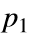,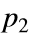,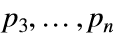. Compute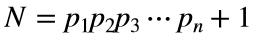. This number N is either prime or composite.
If it is prime, then the original supposition that the primes form a finite set is false. For example, if we assume that the only primes are 2, 3, and 5, then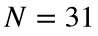, which is prime. Therefore, 31 should be in the set of primes.
If N is composite, then there must be another prime number because N is not divisible by any of the primes in the original set. For example, if we assume the only primes are 2, 3, 5, and 31, then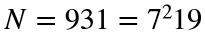. Therefore, 7 and 19 should be in the set as well.
Either way, a contradiction is reached, and the set of primes must be infinite. In other words, we can always add another prime to the set.
Write a function to return the nth Euclid number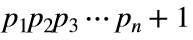as a character string, where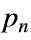is the nth prime. Take the zeroth Euclid number to be 2.

### Solution Stats

50.0% Correct | 50.0% Incorrect
Last Solution submitted on Dec 06, 2022

### Community Treasure Hunt

Find the treasures in MATLAB Central and discover how the community can help you!

Start Hunting!